Ex 2.3

Chapter 2 Class 10 Polynomials
Serial order wise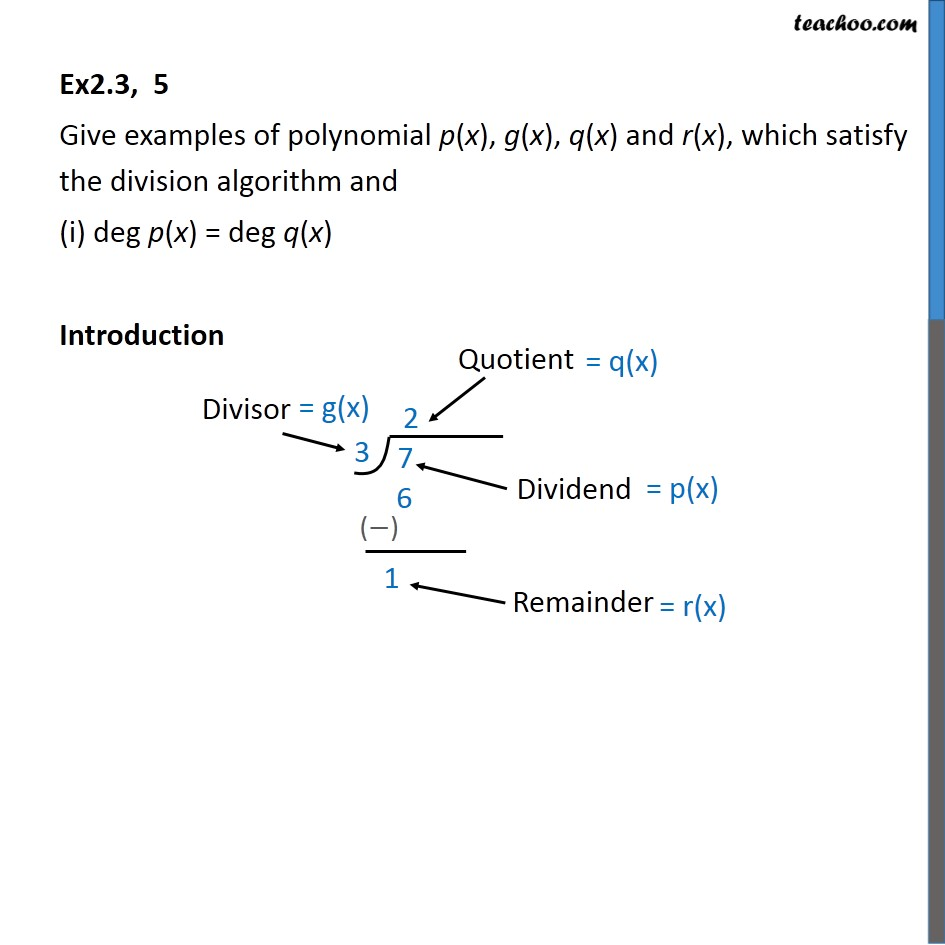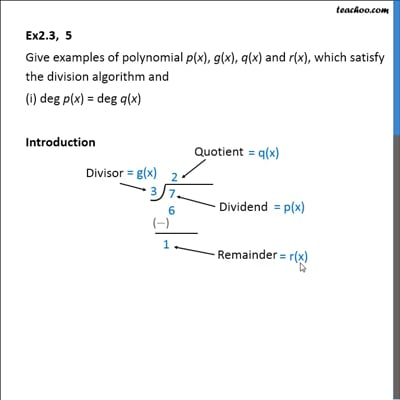This video is only available for Teachoo black users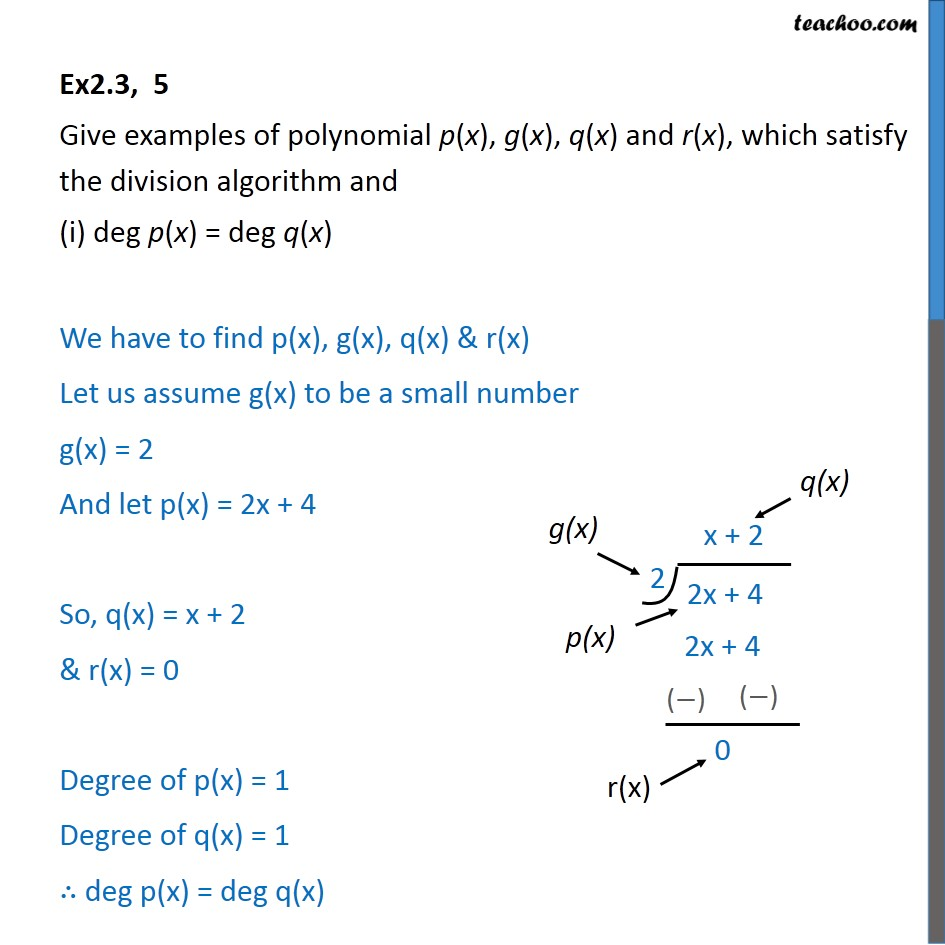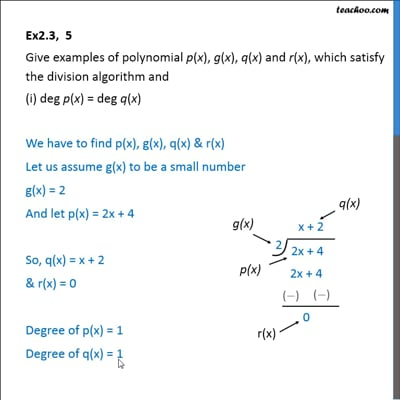This video is only available for Teachoo black users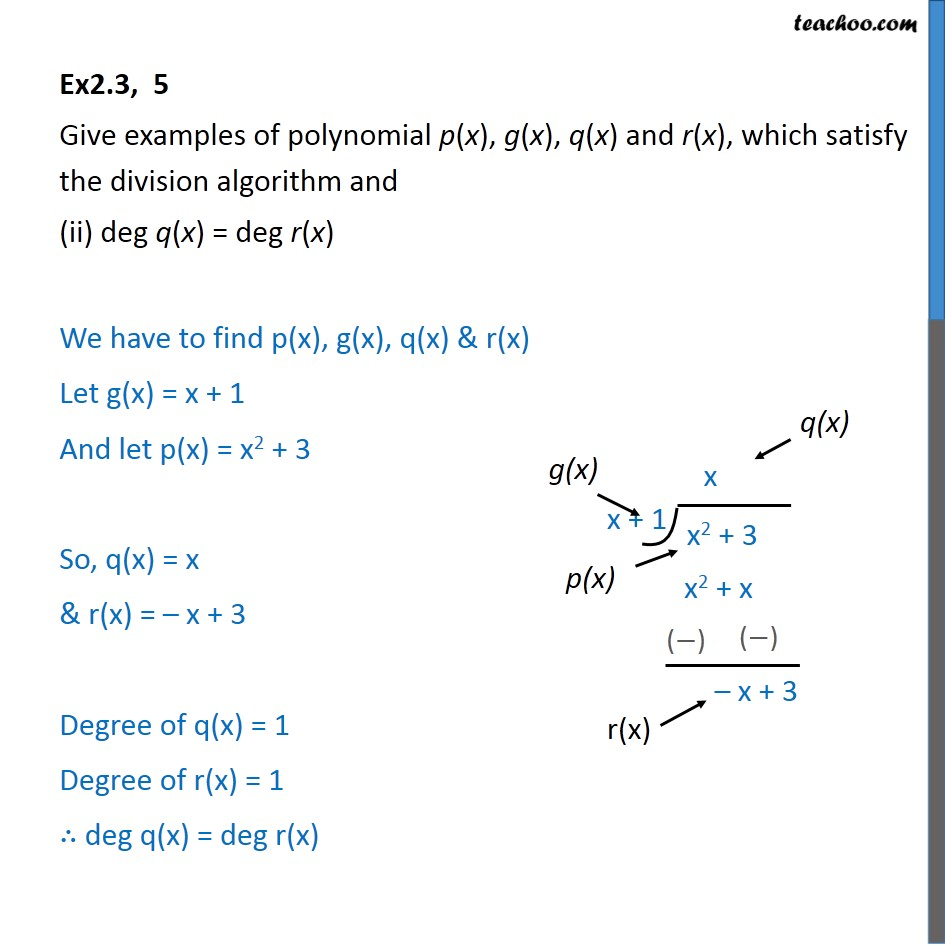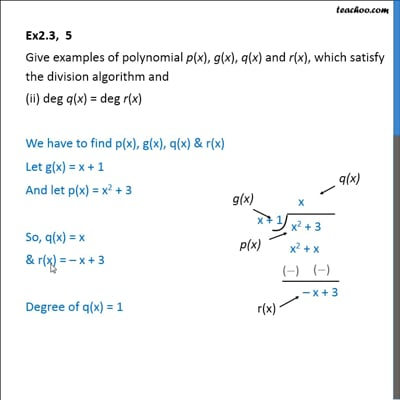This video is only available for Teachoo black users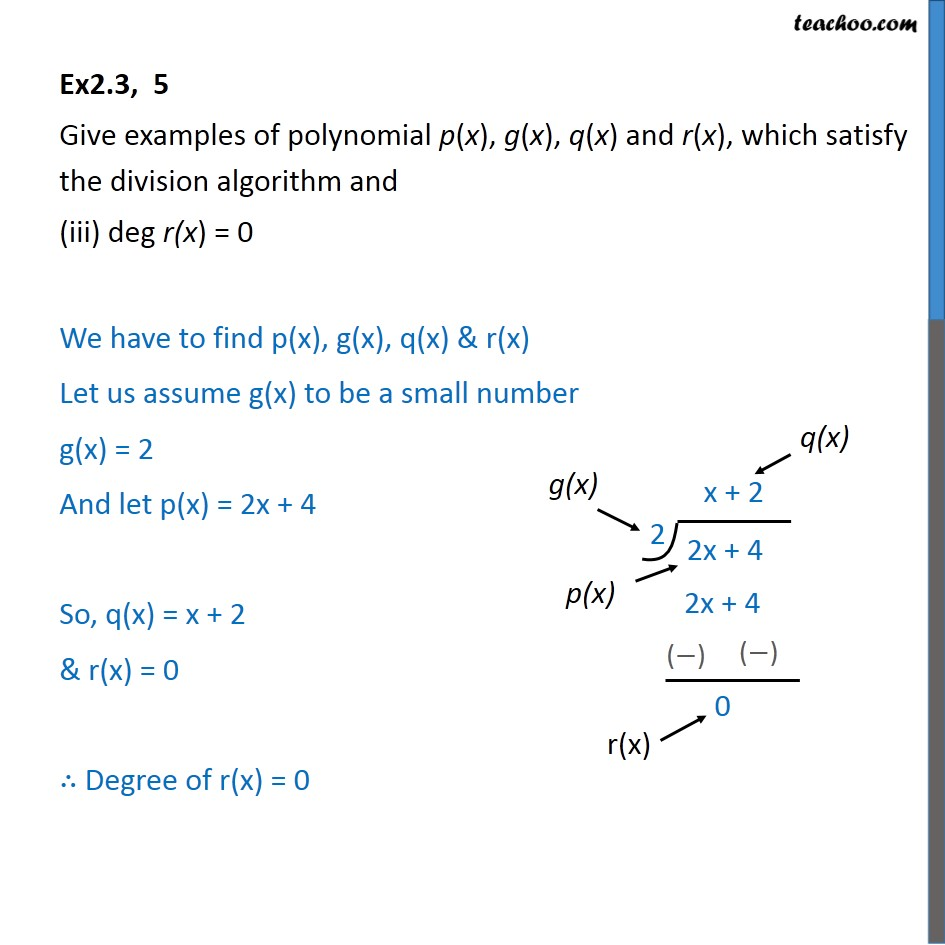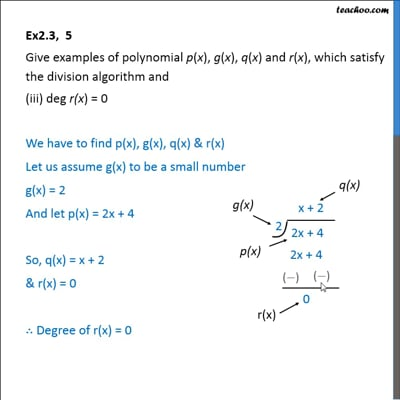This video is only available for Teachoo black users

Maths Crash Course - Live lectures + all videos + Real time Doubt solving!

### Transcript

Ex2.3, 5 Give examples of polynomial p(x), g(x), q(x) and r(x), which satisfy the division algorithm and (i) deg p(x) = deg q(x) Introduction Ex2.3, 5 Give examples of polynomial p(x), g(x), q(x) and r(x), which satisfy the division algorithm and (i) deg p(x) = deg q(x) We have to find p(x), g(x), q(x) & r(x) Let us assume g(x) to be a small number g(x) = 2 And let p(x) = 2x + 4 So, q(x) = x + 2 & r(x) = 0 Degree of p(x) = 1 Degree of q(x) = 1 deg p(x) = deg q(x) Ex2.3, 5 Give examples of polynomial p(x), g(x), q(x) and r(x), which satisfy the division algorithm and (ii) deg q(x) = deg r(x) We have to find p(x), g(x), q(x) & r(x) Let g(x) = x + 1 And let p(x) = x2 + 3 So, q(x) = x & r(x) = x + 3 Degree of q(x) = 1 Degree of r(x) = 1 deg q(x) = deg r(x) Ex2.3, 5 Give examples of polynomial p(x), g(x), q(x) and r(x), which satisfy the division algorithm and (iii) deg r(x) = 0 We have to find p(x), g(x), q(x) & r(x) Let us assume g(x) to be a small number g(x) = 2 And let p(x) = 2x + 4 So, q(x) = x + 2 & r(x) = 0 Degree of r(x) = 0